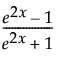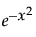Publication date: 07/30/2020

## Hidden Layer Structure

Note: The standard edition of JMP uses only the TanH activation function, and can fit only neural networks with one hidden layer.

The Neural platform can fit one or two-layer neural networks. Increasing the number of nodes in the first layer, or adding a second layer, makes the neural network more flexible. You can add an unlimited number of nodes to either layer. The second layer nodes are functions of the X variables. The first layer nodes are functions of the second layer nodes. The Y variables are functions of the first layer nodes.

Caution: You cannot use boosting with a two-layer neural network. If you specify any non-zero values in the second layer and also specify boosting, the second layer is ignored.

The functions applied at the nodes of the hidden layers are called activation functions. An activation function is a transformation of a linear combination of the X variables. The following activation functions are available:

TanH

The hyperbolic tangent function is a sigmoid function. TanH transforms values to be between -1 and 1, and is the centered and scaled version of the logistic function. The hyperbolic tangent function is:where x is a linear combination of the X variables.

Linear

The identity function. The linear combination of the X variables is not transformed.

The Linear activation function is most often used in conjunction with one of the non-linear activation functions. In this case, the Linear activation function is placed in the second layer, and the non-linear activation functions are placed in the first layer. This is useful if you want to first reduce the dimensionality of the X variables, and then have a nonlinear model for the Y variables.

For a continuous Y variable, if only Linear activation functions are used, the model for the Y variable reduces to a linear combination of the X variables. For a nominal or ordinal Y variable, the model reduces to a logistic regression.

Gaussian

The Gaussian function. Use this option for radial basis function behavior, or when the response surface is Gaussian (normal) in shape. The Gaussian function is:where x is a linear combination of the X variables.